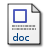# A linear programming approach for the weighted graph matchingproblem

Almohamad, H.A. and Duffuaa, S.O. (1993) A linear programming approach for the weighted graph matchingproblem. Pattern Analysis and Machine Intelligence, IEEE Transactions on, 15.Preview
PDF
18KbMicrosoft Word
26Kb

## Abstract

A linear programming (LP) approach is proposed for the weighted graph matching problem. A linear program is obtained by formulating the graph matching problem in L1 norm and then transforming the resulting quadratic optimization problem to a linear one. The linear program is solved using a simplex-based algorithm. Then, approximate 0-1 integer solutions are obtained by applying the Hungarian method on the real solutions of the linear program. The complexity of the proposed algorithm is polynomial time, and it is O(n 6L) for matching graphs of size n. The developed algorithm is compared to two other algorithms. One is based on an eigendecomposition approach and the other on a symmetric polynomial transform. Experimental results showed that the LP approach is superior in matching graphs than both other methods

Item Type: Article May 1993 Publication Computer College Of Computer Sciences and Engineering > Systems Engineering Dept Almohamad, H.A. and Duffuaa, S.O. 14224 KFUPM ePrints Admin 24 Jun 2008 16:27 12 Apr 2011 13:15

Repository Staff Only: item control page